1. /
2. CBSE
3. /
4. Class 08
5. /
6. Mathematics
7. /
8. NCERT Solutions for Class...

# NCERT Solutions for Class 8 Maths Exercise 4.1

NCERT solutions for Class 8 Maths Practical Geometry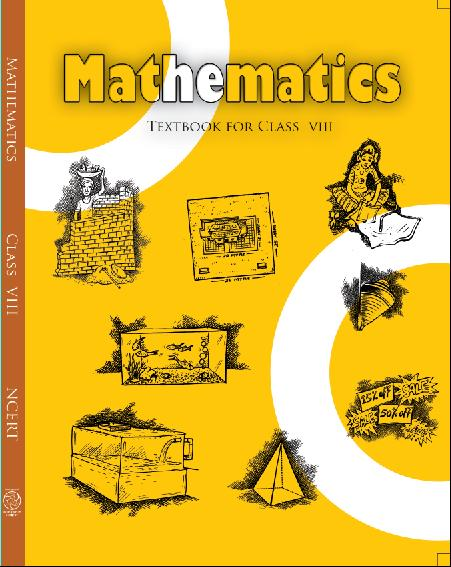## NCERT Solutions for Class 8 Maths Chpater 4 Practical Geometry

Class 8 Maths Exercise 4.1

AB = 4.5 cm, BC = 5.5 cm, CD = 4 cm,

AD = 6 cm, AC = 7 cm

JU = 3.5 cm, UM = 4 cm, MP = 5 cm,

PJ = 4.5 cm, PU = 6.5 cm

(iii) Parallelogram MORE

OR = 6 cm, RE = 4.5 cm, EO = 7.5 cm

(iv) Rhombus BEST

BE = 4.5 cm, ET = 6 cm

Ans.

(i) Given:  AB = 4.5 cm, BC = 5.5 cm,

CD = 4 cm, AD = 6 cm, AC = 7 cm

### Steps of construction: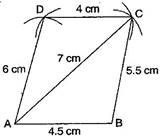(a) Draw AB = 4.5 cm.

(b) Draw an arc taking radius 5.5 cm from point B.

(c) Taking radius 7 cm, draw an another arc from point A which intersects the first arc at point C.

(d) Join BC and AC.

(e) Draw an arc of radius 6 cm from point A and draw another arc of radius 4 cm from point C which intersects at D.

(ii) Given:  JU = 3.5 cm, UM = 4 cm,

MP = 5 cm, PJ = 4.5 cm, PU = 6.5 cm

### Steps of construction: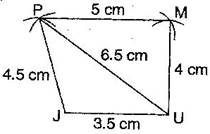(a) Draw JU = 3.5 cm.

(b) Draw an arc of radius 4.5 cm taking centre J and then draw another arc of radius 6.5 cm taking U as centre. Both arcs intersect at P.

(c) Join PJ and PU.

(d) Draw arc of radius 5 cm and 4 cm taking P and U as centres respectively, which intersect at M.

(e) Join Mp and MU.

(iii) Given:  OR = 6 cm, RE = 4.5 cm,

EO = 7.5 cm

To construct: A parallelogram MORE.

### Steps of construction: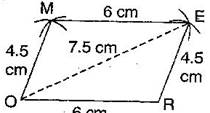(a) Draw OR = 6 cm.

(b) Draw arcs of radius 7.5 cm and radius 4.5 cm taking O and R as centres respectively, which intersect at E.

(c) Join OE and RE.

(d) Draw an arc of 6 cm radius taking E as centre.

(e) Draw another arc of 4.5 cm radius taking O as centre, which intersects at M.

(f) Join OM and EM.

It is required parallelogram MORE.

(iv) Given:  BE = 4.5 cm, ET = 6 cm

To construct: A rhombus BEST.

### Steps of construction: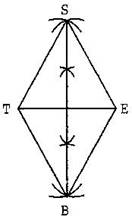(a) Draw TE = 6 cm and bisect it into two equal parts.

(b) Draw up and down perpendiculars to TE.

(c) Draw two arcs of 4.5 cm taking E and T as centres, which intersect at S.

(d) Again draw two arcs of 4.5 cm taking E and T as centres, which intersects at B.

(e) Join TS, ES, BT and EB.

It is the required rhombus BEST.

## NCERT Solutions for Class 8 Maths Exercise 4.1

NCERT Solutions Class 8 Maths Exercise 4.1 PDF (Download) Free from myCBSEguide app and myCBSEguide website. Ncert solution class 8 Mathematics includes text book solutions from Class 8 Maths Book . NCERT Solutions for CBSE Class 8 Maths have total 16 chapters. 8 Maths NCERT Solutions in PDF for free Download on our website. Ncert class 8 solutions PDF and Maths ncert class 8 PDF solutions with latest modifications and as per the latest CBSE syllabus are only available in myCBSEguide.

## CBSE app for Class 8

To download NCERT Solutions for class 8 Social Science, Computer Science, Home Science,Hindi ,English, Maths Science do check myCBSEguide app or website. myCBSEguide provides sample papers with solution, test papers for chapter-wise practice, NCERT solutions, NCERT Exemplar solutions, quick revision notes for ready reference, CBSE guess papers and CBSE important question papers. Sample Paper all are made available through the best app for CBSE students and myCBSEguide website.Test Generator

Create question paper PDF and online tests with your own name & logo in minutes.myCBSEguide

Question Bank, Mock Tests, Exam Papers, NCERT Solutions, Sample Papers, Notes

### 9 thoughts on “NCERT Solutions for Class 8 Maths Exercise 4.1”

1. This is a good way to get solution of math for students

2. my favourite maths

3. This a very good way to find the solution

4. It is easy to do the problems .

5. This is very nice solution it is very easy

6. Nice method to do math…

7. It is very easy sum

8. I can easily complete my exam syllabus using this……

9. I can score my marks at exam because it’s interesting.